Browse by Topic
Related Topics

# Nine Cool Points on the Complex Plane

Pick some points at random. What can be said about them? What curves go through them? What polygons and polynomials can be made from them? Deep mathematics lurks behind these questions, but the answers can be explored just by moving points around within some Wolfram Demonstrations.

Simply by moving points you can see deep mathematics in action.

For example, “Five Points Determine a Conic Section” (Ed Pegg Jr and Paul Abbott) uses a matrix determinant on five points to produce an equation going through all five points.

The following matrix calculates the ellipse. You don’t necessarily need to know how to calculate a determinant yourself. Similarly, while playing a computer game, you don’t need to know how rotation matrices work, even though thousands or millions of them get calculated every second you play.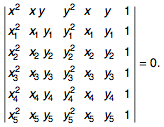When a point is moved, a picture is made after the conic section matrix calculations. With nine points, a more complicated equation known as an elliptic curve (or cubic) can be calculated. Try moving the points of the “Nine-Point Cubic” Demonstration (Ed Pegg Jr) to see the various shapes of elliptic curves.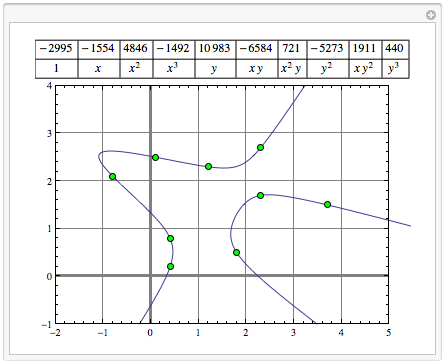The equation of the cubic through the nine points can be computed with the following determinant. I’m not sure who discovered this matrix first, but it wasn’t me. I knew three things: the cubic equation, the conic section matrix, and that nine points were involved. I tried setting up the same sort of matrix, and it worked.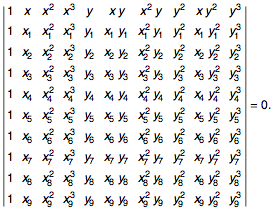The nine points above are of the form (a,b), but that’s not the only way to express points. The other common way is for each point to represent a unique complex number of the form a+b i, where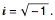By “Factoring Gaussian Integers” (Izidor Hafner), the roots of complex numbers corresponding to integers can be calculated and plotted on the complex plane.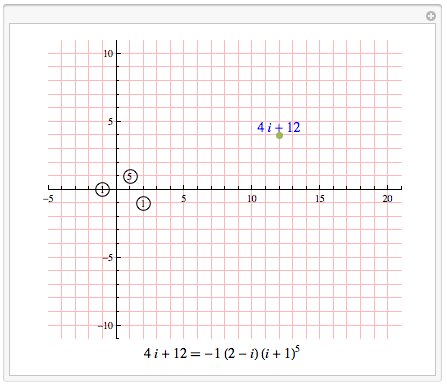When a number appears in a factor circle, that represents the exponent of that particular complex number. It turns out that the “Powers of Complex Points” (Ed Pegg Jr) have some fascinating patterns. Below, the Voronoi regions corresponding to each Gaussian integer power are displayed.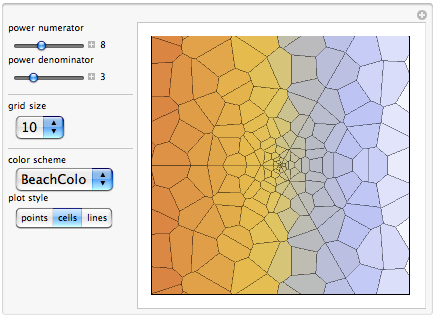Let v1, v2, v3, and v4 be complex numbers of the form vn=a+i b. Similarly, let u1, u2, u3, and u4 be complex numbers of the form un=a+ i b. A complex polynomial can be presented in two forms: the g(z) form that is defined by the complex roots and the f(z) form that is defined by the complex coefficients.

g(z) =(zv1)(zv2)(zv3)(zv4)

f(z)=u0+u1z+u2 z2+u3 z3+u4 z4

Both are equivalent, with f(z) = g(z). To go from g(z)-> f(z), multiply. Going the other way, f(z) -> g(z), is harder. The Mathematica functions Solve[] and NRoots[] can do it. A more classic method, Newton’s method, uses an initial guess w0 and the recursion wn+1=wnf(wn])/f’(wn) to find roots of the equation f(z). In the complex plane, equations with multiple roots show fractal behavior within the “Complex Newton Map” (Ed Pegg Jr). The points are the four vn roots and the four colors indicate the root that is reached when the initial guess is w0.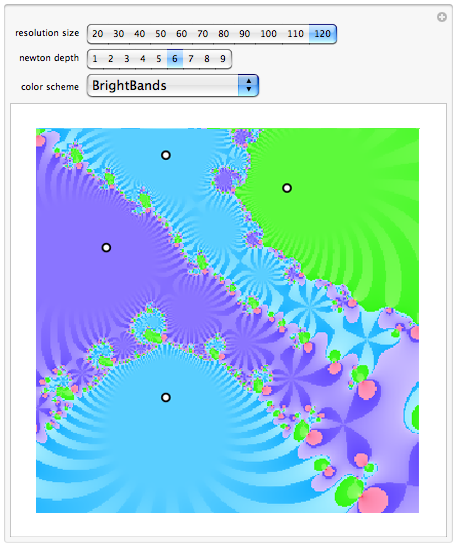For z=a+b i, let Re(z) =a, and Im(z) =b. These are the real and imaginary parts of a complex number. Next, consider “Complex Polynomials” (Ed Pegg Jr) of the form g(z) as defined in the paragraph above. What does g(z) look like when plotted? In a two-dimensional plot, a solid green line can be used when Re(g(z))=0, and a dashed green line when Im(g(z))=0. At the roots, the green lines cross, indicating that both the real and imaginary parts equal zero. The red lines plot out the first derivative g’(z), with only three roots. The next derivative uses blue lines.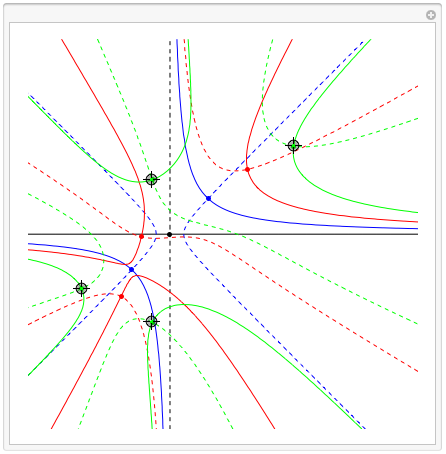Notice how the red points above are within an area bounded by the green points. For any complex polynomial, the roots bound the derivative’s roots. This is the “Lucas–Gauss Theorem” (Bruce Torrence), and is shown below with a series of eight derivatives. No matter how the points are moved, a series of nested polygons will result.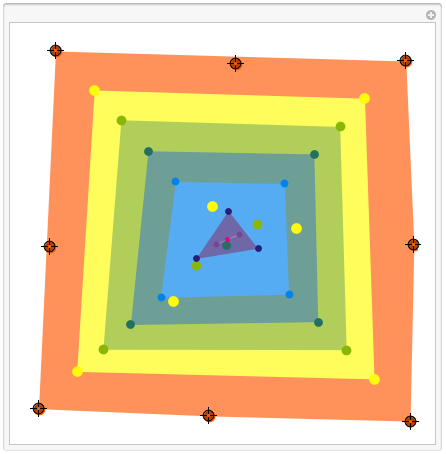Here is a math problem: for a triangle ABC, find the foci of the ellipse that is tangent to the midpoints of each side. Sounds tricky, doesn’t it?

Here is the solution: Let ABC be complex roots of g(z). The foci are the roots of g’(z). Done. This is “Marden’s Theorem” (Bruce Torrence), which has been called the most marvelous theorem in mathematics. As a side note, the roots of a derivative are known as the critical points.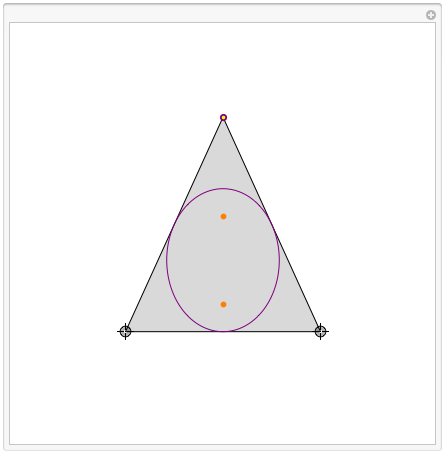Complex mathematics solved a tricky problem instantly, revealing some of its power, but not everything is entirely understood. Suppose all the roots of a polynomial are bounded by the unit disk. Take one of the roots and draw a disk of radius one around it. Does that disk always contain one of the critical points—one of the derivative roots? Amazingly, no one knows yet. So far, every gray disk has always contained an orange dot, no matter how the points are moved around. This is known as “Sendov’s Conjecture” (Bruce Torrence).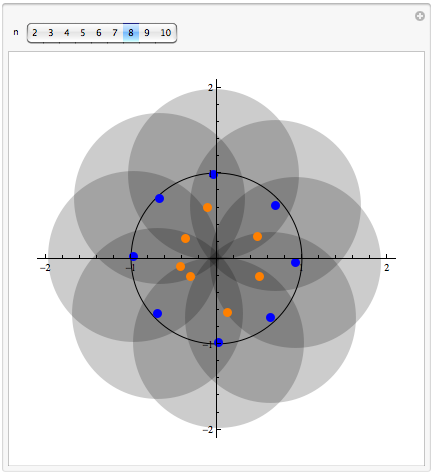In this example, if you move the points around and watch the general behavior, you pretty much know as much as anyone about the mathematics, since this problem is unsolved.

Even though the math might be hard in all of these examples, you can quickly gain intuition on the effects of moving a point. The mathematics behind the average video game is even harder. Going up against an average 10-year-old in a computer game, I’d probably lose. The video-card part of his or her brain is able to react faster. However, I’d do better if we went right into the mathematics and unplugged the kid’s superior video-processing talents. Which is the better way for a kid to learn? The answer to that is likely complex.